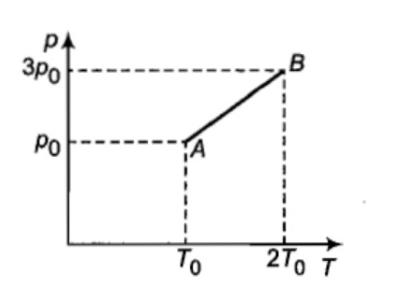The pressure versus temperature graph of an ideal gas is as shown in the figure.  The density of the gas at point A is ${\rho }_{0}$.  The density at B will be:(1) $\frac{3}{4}{\rho }_{0}$          (2) $\frac{3}{2}{\rho }_{0}$            (3) $\frac{4}{3}{\rho }_{0}$              (4) 2 ${\rho }_{0}$